Published

# Temperature Sensor and Anomaly Detector

Well sometimes the fridge remains open due to negligence, which could spoil food. Through IoT technology we are going to solve our problem.

IntermediateFull instructions provided3 hours691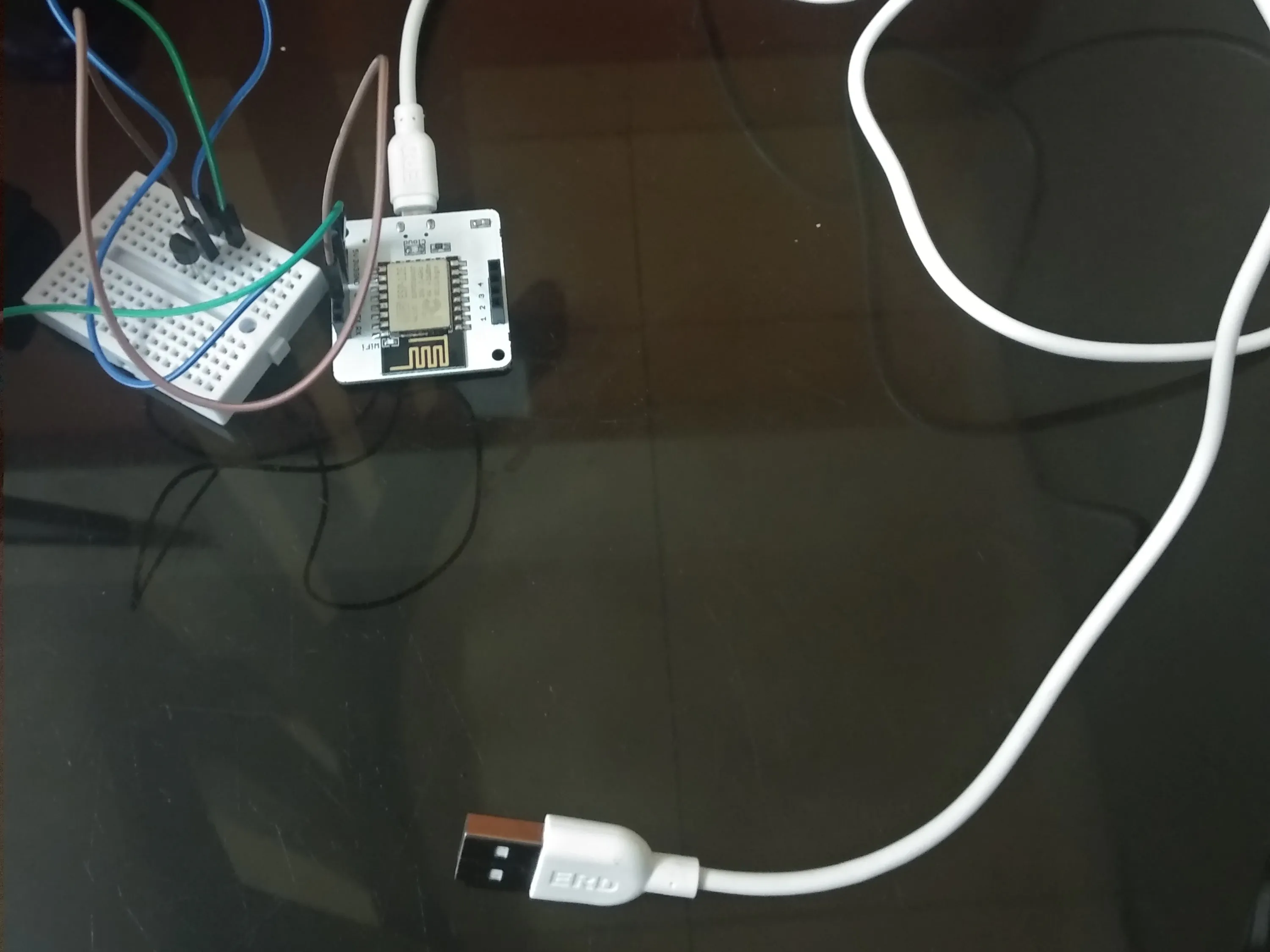## Things used in this project

### Hardware componentsJumper wires (generic)
×3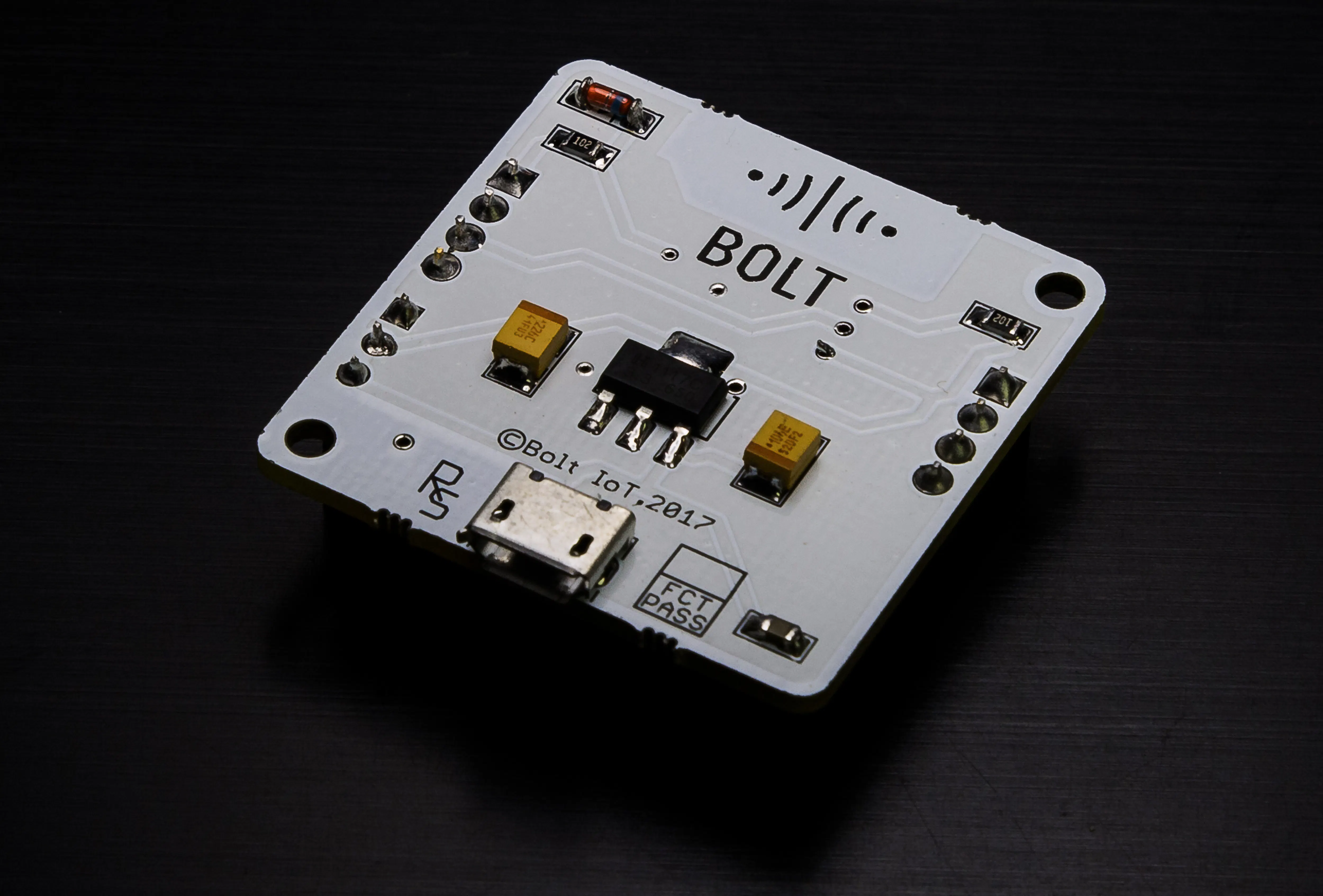Bolt IoT Bolt WiFi Module
×1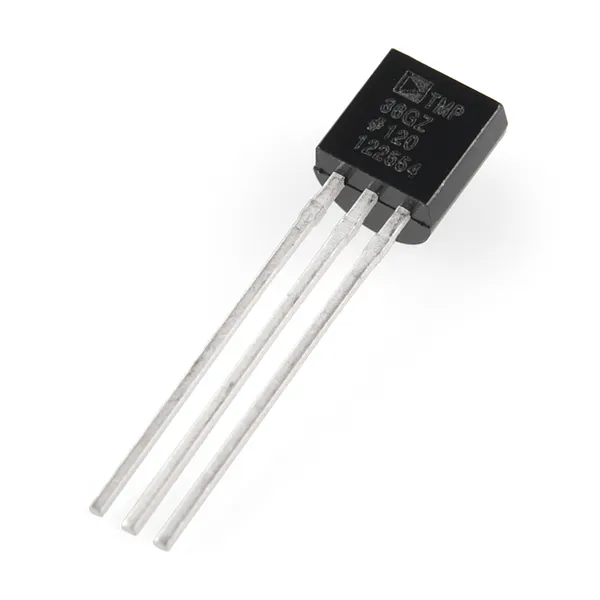Temperature Sensor
×1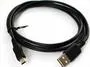USB-A to Mini-USB Cable
×1×1

### Software apps and online servicesBolt IoT Bolt Cloud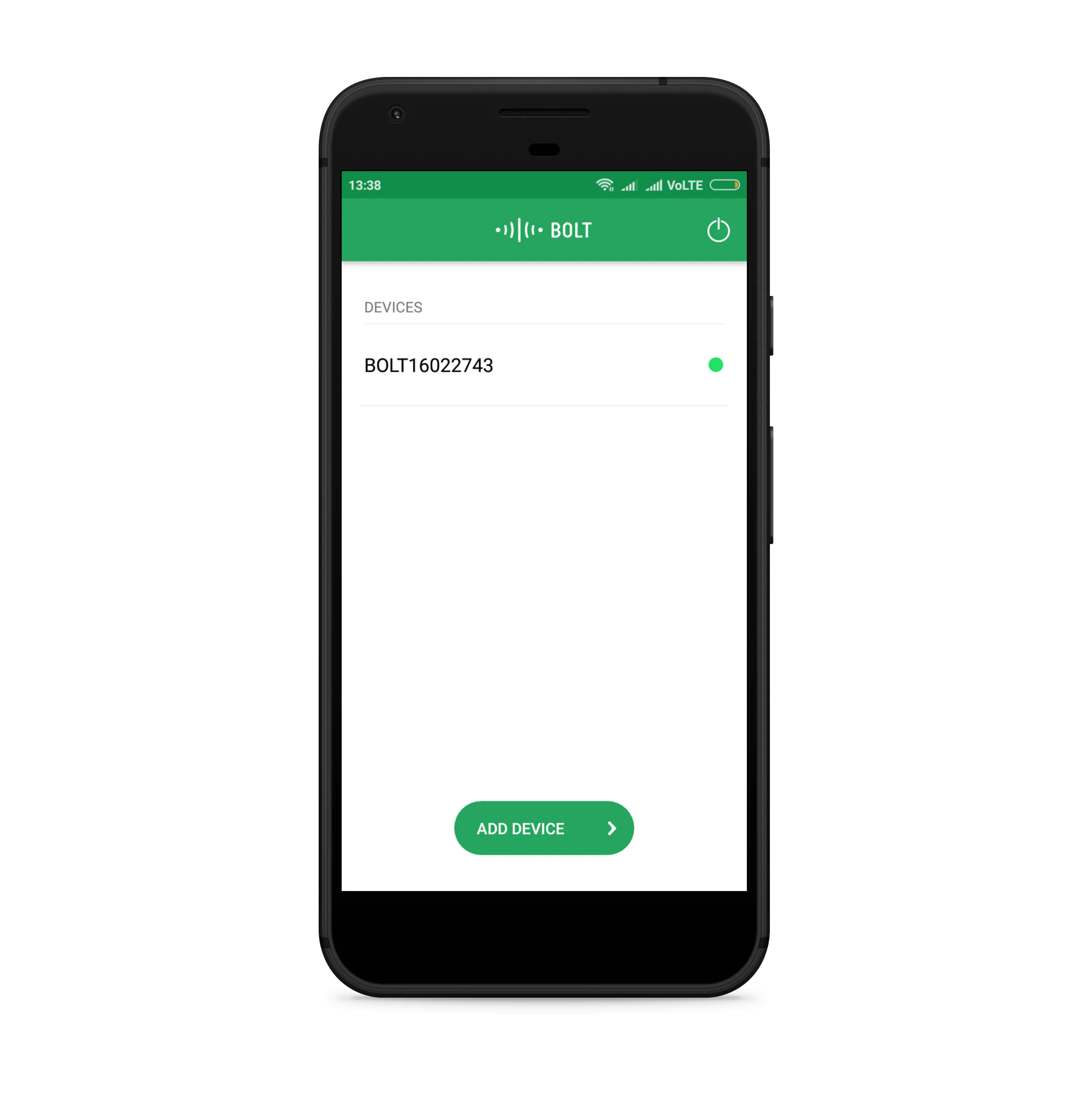Bolt IoT Android AppTwilio SMS Messaging API

## Schematics

### The Connection

Temperature Sensor and Bolt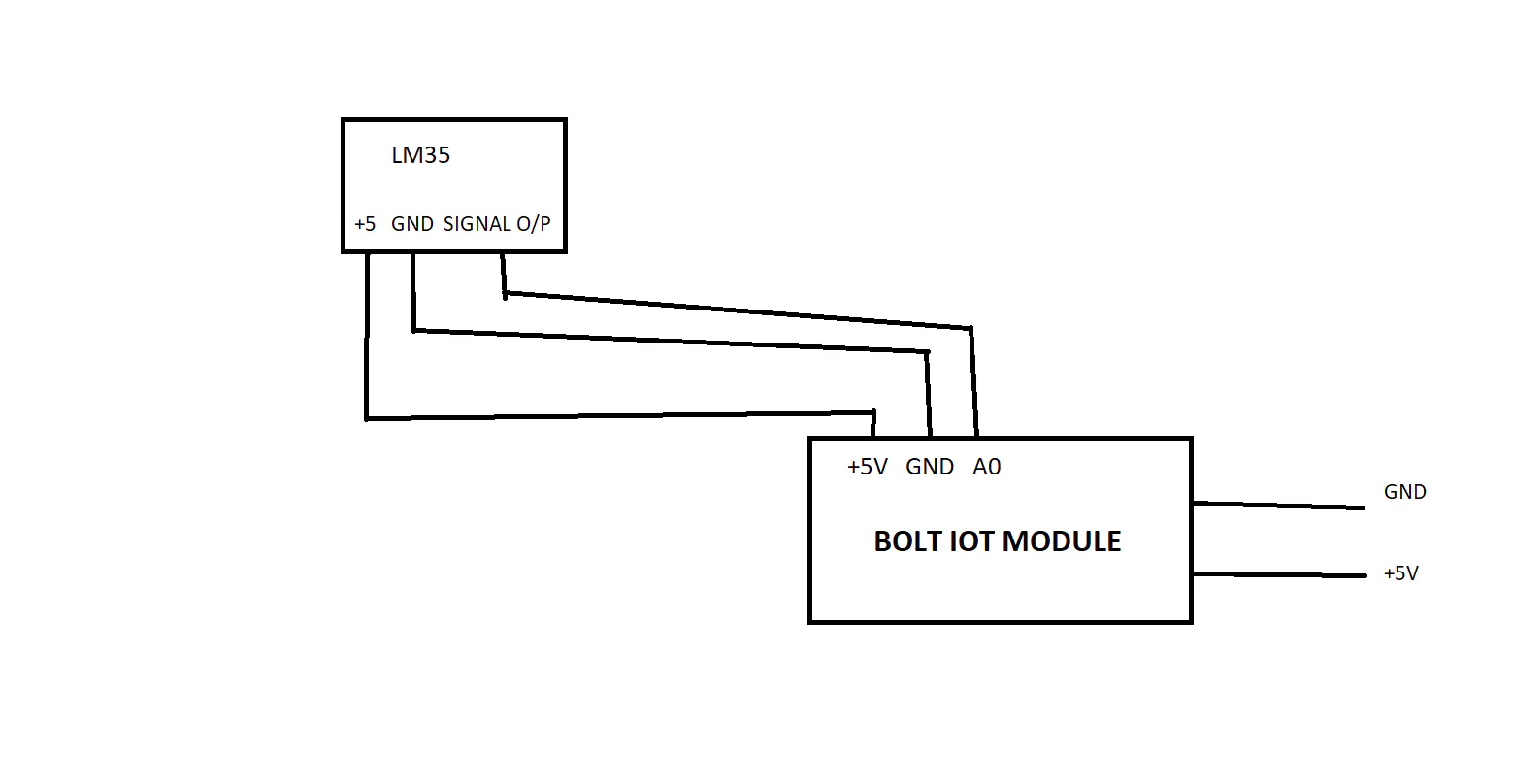## Code

### Anomaly Detection

Python
This has the whole cod needed to create the project
```import conf, json,time, requests, statistics, math
from boltiot import Sms, Bolt

def data_get(hdata1, frame, factor):

if len(hdata)<frame :
return None
if len(hdata)>frame :
del hdata[0:len(hdata)-frame]

Mean = statistics.mean(hdata)

Variance= 0

for data in hdata :
Varianace= Variance + math.pow((data-Mean),2)

zn = factor * math.sqrt(Variance/frame)

hbound = hdata[frame-1]+zn
lbound = hdata[frame-1]-zn
return[hbound,lbound]

minlimit = 5
maxlimit = 11

mybolt = Bolt(conf.api, conf.id)
sms = Sms(conf.ssid, conf.token, conf.ton, conf.fromn)
hdata=[]

while True:
print ("THE DATA INCOMING", data)
if data['success'] != 1:
print("There was an error while retriving the data.")
print("This is the error:" +data['value'])
time.sleep(10)
continue

print ("The value is "+data['value'])
sensor=0
try:
sensor = int(data['value'])
except Exception as e:
print("Error. Try again",  e)
continue

bound = data_get(hdata, conf.frame, conf.factor)
if not bound :
reqbound= conf.frame -len(hdata)
print ("No. of more points needed is" , reqbound )
hdata.append(int (data['value']))
time.sleep(10)
continue

try :

temp = sensor/10.24
if temp > maxlimit:

print ("The Temperature value increased suddenly.Somebody opened the fridge. Sending sms.")
print ("The Current temperature is: "+str(temp)+" °C")
response = sms.send_sms("Alert ! Someone has opened the fridge door. The temperature is :"+ str(temp)+ "°C")
print("This is the response ",response)

elif temp > minlimit or temp < maxlimit:
print("The fridge is cooling and tablet is safe")

print (" The Current temperature is:" +str(temp)+ "°C")
response = sms.send_sms("The fridge is cooling and tablet is safe")
print("This is the response",response)
hdata.append(sensor)

except Exception as e:
print ("Error",e)
time.sleep(10)
```

### Regression Graph

Python
Bolt Cloud
```setChartLibrary('google-chart');
setChartTitle('Polynomial Regression');
setChartType('predictionGraph');
setAxisName('time_stamp','temp');
mul(0.0977);
plotChart('time_stamp','temp');
```

### Configuration file

Python
Basic stuff
```SID = 'You can find SID in your Twilio Dashboard'
AUTH_TOKEN = 'You can find  on your Twilio Dashboard'
FROM_NUMBER = 'This is the no. generated by Twilio. You can find this on your Twilio Dashboard'
TO_NUMBER = 'This is your number. Make sure you are adding +91 in beginning
API_KEY = 'This is your Bolt Cloud accout API key'
DEVICE_ID = 'This is the ID of your Bolt device'
```

## Credits

### Dhruvajit Ghosh

9 projects • 1 follower